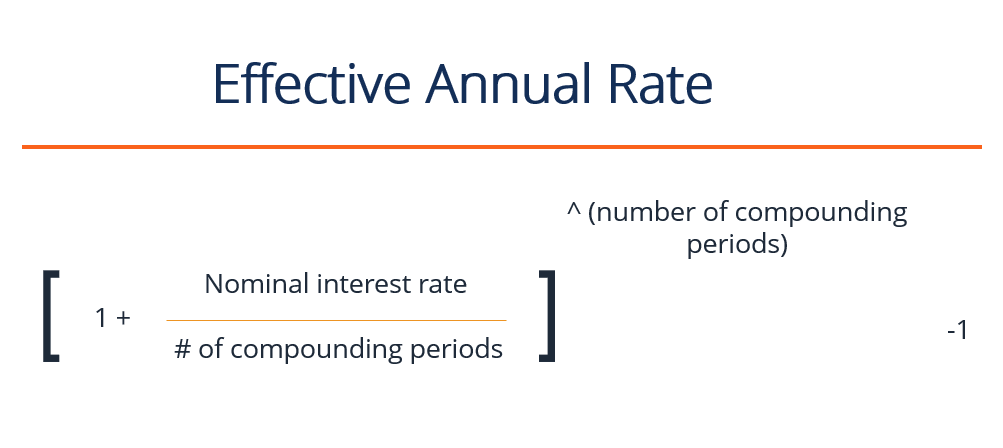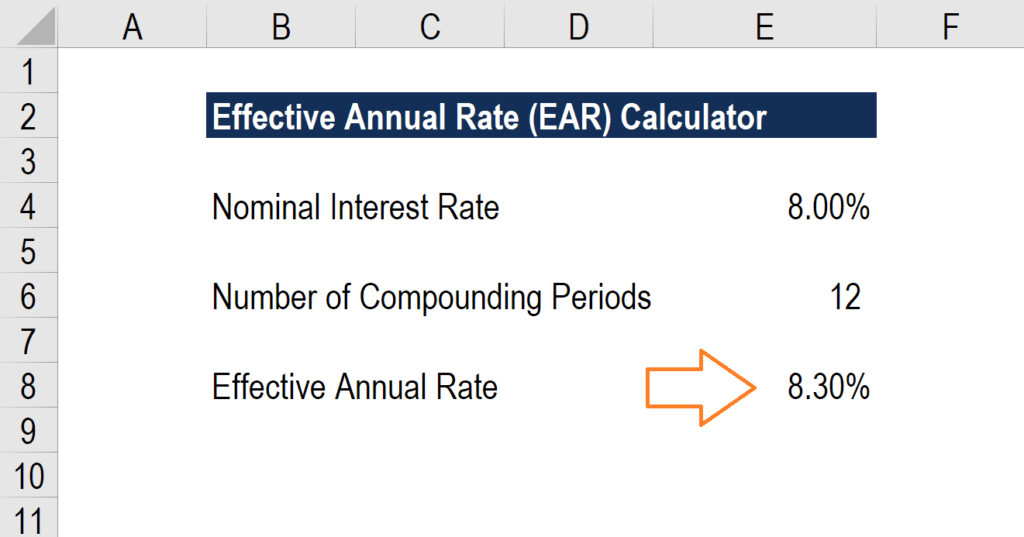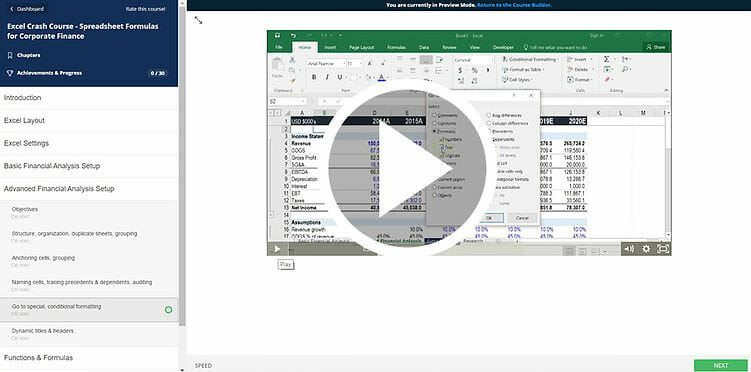# Effective Annual Rate

Actual rate of return received by investors or the actual interest rate paid by borrowers.

## What is the Effective Annual Rate?

The Effective Annual Rate (EAR) is the rate of interest actually earned on an investment or paid on a loan as a result of compounding the interest over a given period of time. It is usually higher than the nominal rate and is used to compare different financial products that calculate annual interest with different compounding periods – weekly, monthly, yearly, etc.  Increasing the number of compounding periods makes the effective annual interest rate increase as time goes by.The effective annual rate is normally higher than the nominal rate because the nominal rate quotes a yearly percentage rate regardless of compounding. Increasing the number of compounding periods increases the effective annual rate as compared to the nominal rate. To spin it in another light, an investment that is compounded annually will have an effective annual rate that is equal to its nominal rate. However, if the same investment was instead compounded quarterly, the effective annual rate would then be higher.

### What is the Formula for the Effective Annual Rate?

The formula for the EAR is:

Effective Annual Rate = (1 + (nominal interest rate / number of compounding periods)) ^ (number of compounding periods) – 1

For example:

Union Bank offers a nominal interest rate of 12% on its certificate of deposit to Mr. Obama, a bank client. The client initially invested \$1,000 and agreed to have the interest compounded monthly for one full year. As a result of compounding, the effective interest rate is 12.683%, in which the money grew by \$126.83 for one year, even though the interest is offered at only 12%.

### Effective Annual Rate Calculator

Below is a screenshot of CFI’s free effective annual rate (EAR) calculator.

As you can see in the example above, a nominal interest rate of 8.0% with 12 compounding periods per year equates to an effective annual percentage rate (EAPR) of 8.3%.### What is a Nominal Interest Rate?

A nominal interest rate is a stated rate indicated by a financial instrument that is issued by a lender or guarantor. This rate is the basis for computation to derive the interest amount resulting from compounding the principal plus interest over a period of time.  In essence, this is the actual monetary price that borrowers pay to lenders or that investors receive from issuers.

### What is a Compounding Period?

A compounding period is the time period after which the outstanding loan or investment’s interest is added to the principal amount of said loan or investment. The period can be daily, weekly, monthly, quarterly, or semi-annually, depending on the terms agreed upon by the parties involved.  As the number of compounding periods increases so does the amount of interest earned or paid on the money used. Quarterly compounding produces higher returns than semi-annual compounding, while monthly compounding generates more than quarterly, and daily compounding generates more than monthly.### How Important is the Effective Annual Rate in Business?

The effectual annual interest rate is a useful way of evaluating the actual return on investment and ascertaining the interest expense paid on a loan. Borrowers need to have a solid understanding of the impact cost of debt has on their business, as it will impact their profitability and solvency.

A higher interest expense lowers the interest coverage ratio for a company, which could reduce its ability to service debt in the future. Additionally, the higher interest expense will lower net income and profitability for the company (all else being equal).

On the flip side, investors will benefit if the effective interest rate is greater than the nominal rate offered by the issuer. They also use this rate to compare various investment portfolios by using different compounding periods to make an effective decision.

### More learning

• Weighted Average Cost of Capital (WACC)
• EBITDA
• Basis Points (bps)
• XIRR vs IRR
• Investment Banking
• Financial Modeling

### Financial Analyst Training

Get world-class financial training with CFI’s online certified financial analyst training program!

Gain the confidence you need to move up the ladder in a high powered corporate finance career path.

Learn financial modeling and valuation in Excel the easy way, with step-by-step training.